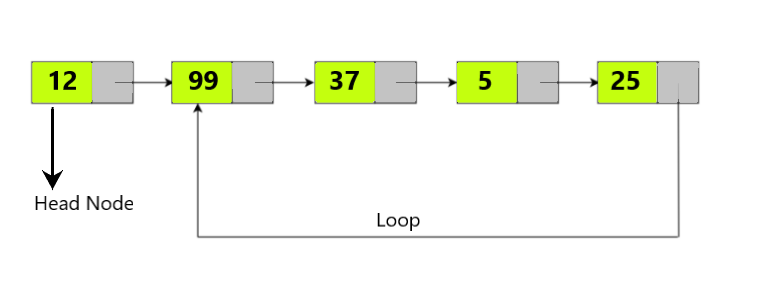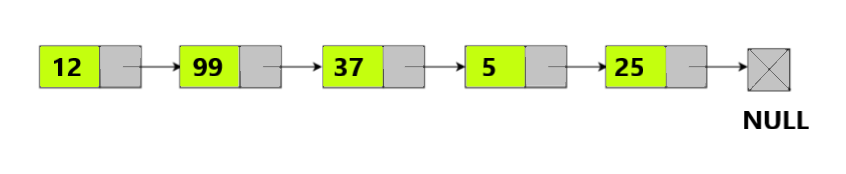# Detect and Remove Loop in a Linked List

In this article, we will look at an interesting problem related to Linked List . ‘Given a Single Linked List, Remove Loop if there exists any’. We will look at different ways to solve this problem and analyze the complexities of our approach.

Now, To Remove a Loop in a Linked List, we need to first Detect a Loop in the linked list. If a Loop exists we remove it with our logic and print the elements of the list. So having a Loop in a Linked List means there is no such node having a NULL pointer or reference. In simpler terms, the Linked list has no end. Let us understand this with an example.Here, we have a Linked List with 5 nodes, Node 12 is the Head node. We can see a Loop exists in the list connecting nodes 25 and 99. So we need to Remove this Loop such that the last node (25) points to NULL. After removal the List should look like this:We can see the last node now points to NULL and the loop no longer exists. Now, let us look at different approaches to solve the problem.

Note: If the Loop starts from any other node except the last node we need to change its reference to NULL to remove the loop.

## Approach 1 (Using Hashing Concept)

• In this approach we will hash or store the references of each node of the Linked List in a Unordered Set or Hash-Set in Java, which contains Unique elements.
• So, we will traverse the list node by node add it to our Set, if not present. We will have a Pointer/Variable prev which will hold the previous node reference and every time we add the node to the Set, we update the prev to Current Node’s reference or address.
• When we encounter a node pointing to a node already present in the Set, we know that we encountered a loop so the set will already have the node when we try to add it.
• Hence in that case we do not add the node again, we make the last node or prev point to NULL. Thereby, removing the loop in the linked list.

Let us look at the implementation of above in java:

Output:

Time Complexity: We do a single traversal of the Linked List until we get the loop so the complexity is O(n).

Space Complexity: We use a Hash-Set which at most stores all the nodes of the Linked List, so the space complexity is O(n), for n nodes in list.

## Approach 2 (Floyd’s Cycle Detection Algorithm)

• In this approach, we use Floyd’s Cycle Detection Method to detect loop/cycle in our linked list. We use two pointers: One Slow and Another Fast Pointer. We move the slow pointer by one position and the fast pointer by two positions. If they meet at a point then we can say Loop is detected.
• Now after detecting loop we have two cases to consider: 1. If Fast Pointer or Loop is Detected at Head Node. 2. If Fast Pointer is at any other node.
• If the Loop is detected at Head Node or the fast pointer is at head node, we move the Slow pointer until it reaches the node before the head node or the node where loop begins and make its reference to NULL.
• For the 2nd  Case, we bring the Slow to position of the head of list. We then continue moving the slow and the fast pointer until slow.next = fast.next . We then make the Fast pointer reference as NULL, thus removing the loop.

Now let us have a quick look at the implementation in java:

Output:

So we have implemented the code for the same example discussed above. We can see the nodes where the loop is detected and removed. Now let us have a quick look at the Complexities for this approach.

Time Complexity: The time complexity is O(n), since we traverse all nodes of the list before we detect the cycle/loop.

Space Complexity: This approach is efficient in terms of space required which is O(1), since we do not use any auxiliary space instead we just used two pointers.

So that’s it for the article you can try implementing the code with different examples. Let us know your suggestions or doubts in the comment section below.